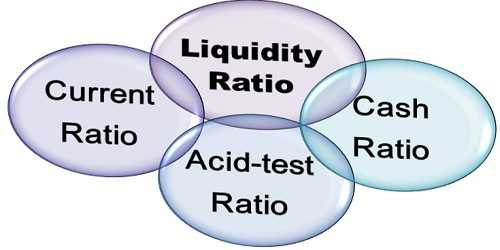# Liquidity Ratios

Liquidity Ratios

In accounting, the term liquidity is defined as the ability of a company to meet its financial obligations as they come due. Liquidity Ratios measure the firms’ ability to pay off current dues i.e., repayable within a year. Liquidity ratios are otherwise called as Short Term Solvency Ratios. The liquidity ratio, then, is a computation that is used to measure a company’s ability to pay its short-term debts.

The important liquidity ratios are

1. Current Ratio
2. Liquid Ratio
3. Absolute Liquid Ratio

These ratios analyze the ability of a company to pay off both its current liabilities as they become due as well as their long-term liabilities as they become current.

Current Ratio

This ratio is used to assess the firm’s ability to meet its current liabilities. The relationship of current assets to current liabilities is known as current ratio. The ratio is calculated as:

Current Ratio = Current Assets / Current Liabilities

Current Assets are those assets, which are easily convertible into cash within one year. This includes cash in hand, cash at bank, sundry debtors, bills receivable, short term investment or marketable securities, stock and prepaid expenses.

Current Liabilities are those liabilities which are payable within one year. This includes bank overdraft, sundry creditors, bills payable and outstanding expenses.

Liquid Ratio

This ratio is used to assess the firm’s short term liquidity. The relationship of liquid  assets to current liabilities is known as liquid ratio. It is otherwise called as Quick ratio or Acid Test ratio. The ratio is calculated as:

Liquid Ratio = Liquid Assets / Current Liabilities

A liquid asset means current assets less stock and prepaid expenses.

Absolute Liquid Ratio

It is a modified form of liquid ratio. The relationship of absolute liquid  assets to liquid liabilities is known as absolute liquid ratio. This ratio is also called as ‘Super Quick Ratio’.  The ratio is calculated as:

Absolute Liquid Ratio = Absolute Liquid Assets / Liquid Liabilities

An absolute liquid asset means cash, bank, and short term investments. Liquid liabilities mean current liabilities less bank overdraft.